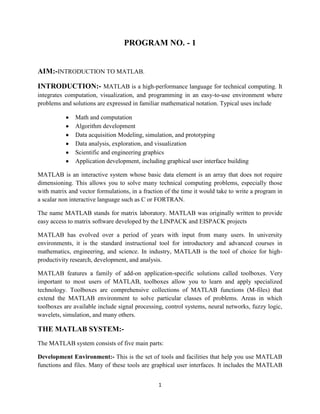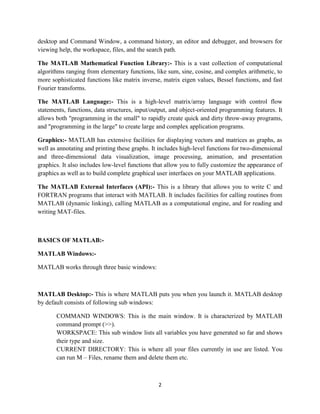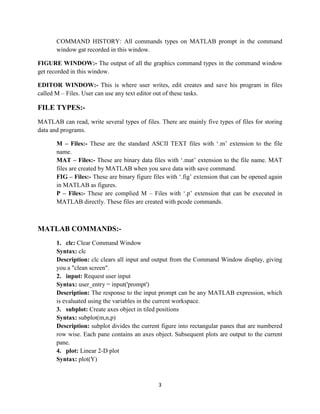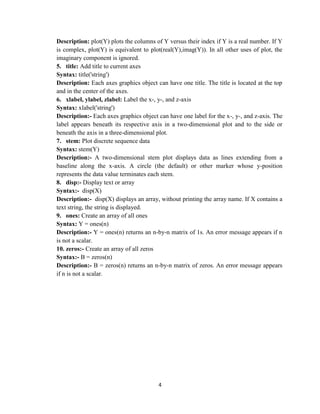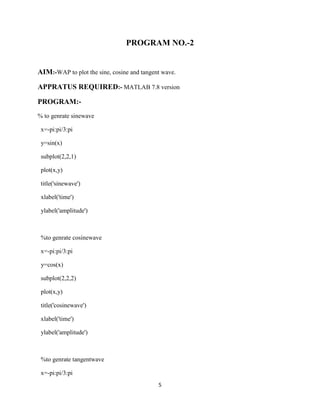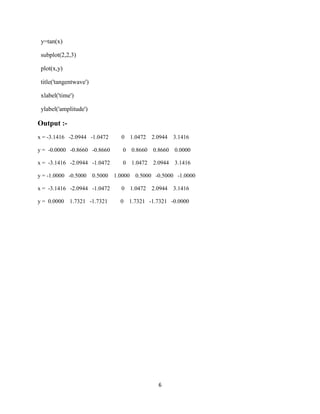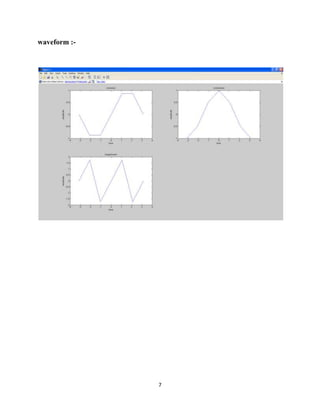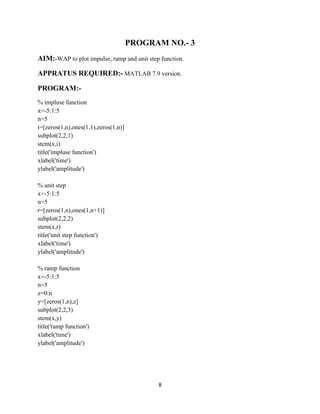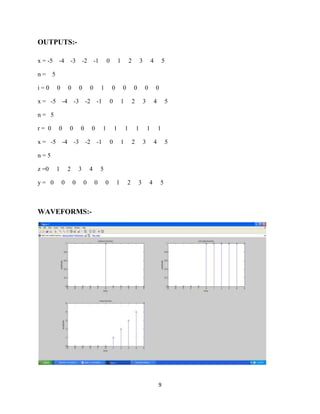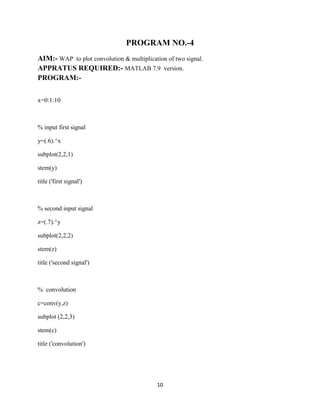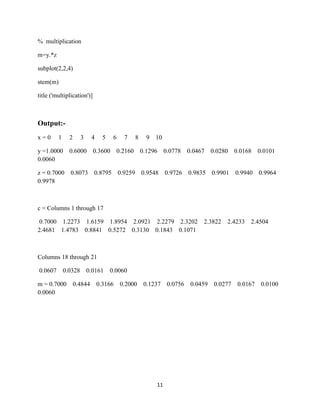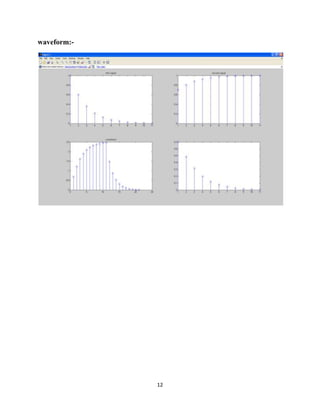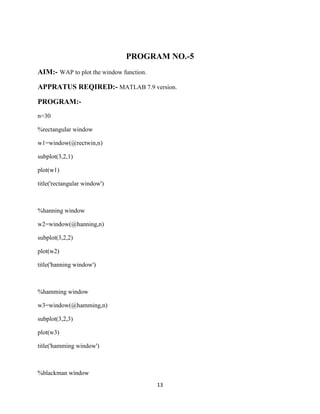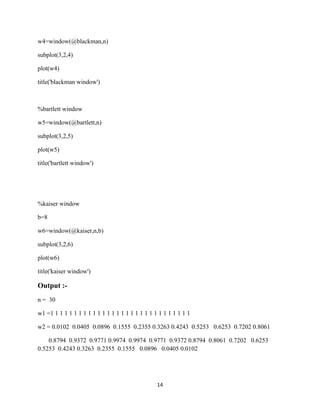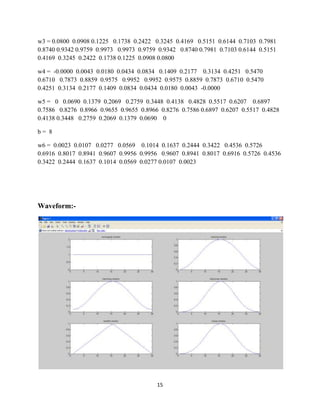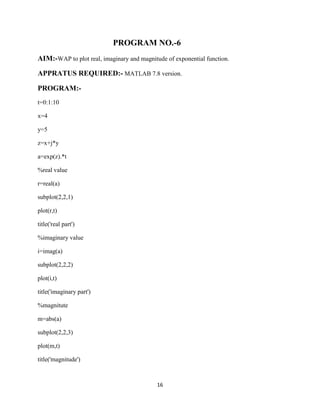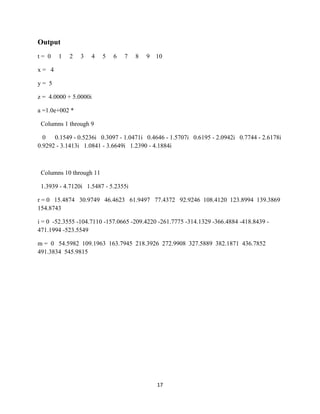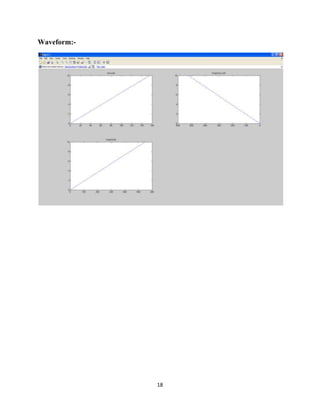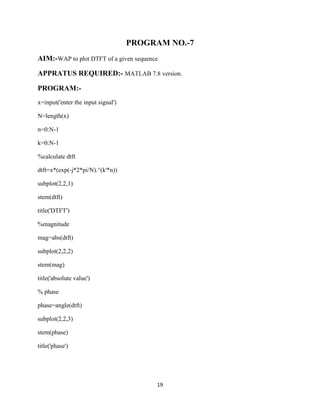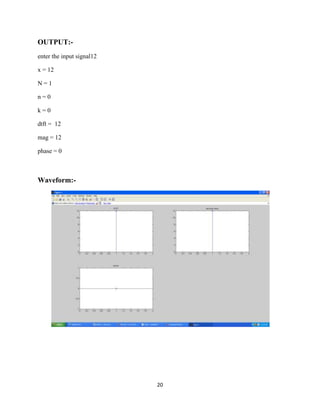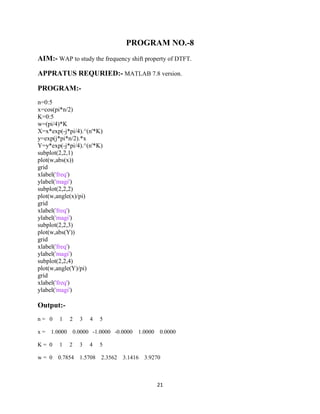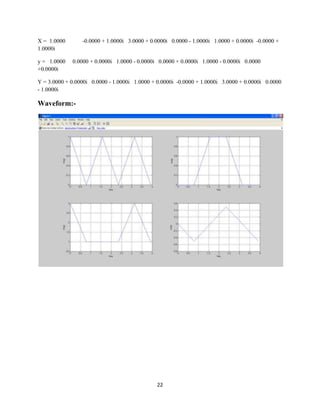Próximo SlideShare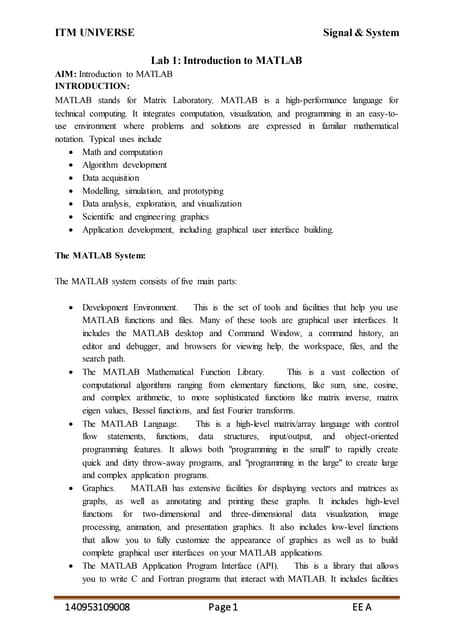KEVIN MERCHANT DOCUMENT
Cargando en ... 3
1 de 23

### Dsp file

1. 1 PROGRAM NO. - 1 AIM:-INTRODUCTION TO MATLAB. INTRODUCTION:- MATLAB is a high-performance language for technical computing. It integrates computation, visualization, and programming in an easy-to-use environment where problems and solutions are expressed in familiar mathematical notation. Typical uses include Math and computation Algorithm development Data acquisition Modeling, simulation, and prototyping Data analysis, exploration, and visualization Scientific and engineering graphics Application development, including graphical user interface building MATLAB is an interactive system whose basic data element is an array that does not require dimensioning. This allows you to solve many technical computing problems, especially those with matrix and vector formulations, in a fraction of the time it would take to write a program in a scalar non interactive language such as C or FORTRAN. The name MATLAB stands for matrix laboratory. MATLAB was originally written to provide easy access to matrix software developed by the LINPACK and EISPACK projects MATLAB has evolved over a period of years with input from many users. In university environments, it is the standard instructional tool for introductory and advanced courses in mathematics, engineering, and science. In industry, MATLAB is the tool of choice for high- productivity research, development, and analysis. MATLAB features a family of add-on application-specific solutions called toolboxes. Very important to most users of MATLAB, toolboxes allow you to learn and apply specialized technology. Toolboxes are comprehensive collections of MATLAB functions (M-files) that extend the MATLAB environment to solve particular classes of problems. Areas in which toolboxes are available include signal processing, control systems, neural networks, fuzzy logic, wavelets, simulation, and many others. THE MATLAB SYSTEM:- The MATLAB system consists of five main parts: Development Environment:- This is the set of tools and facilities that help you use MATLAB functions and files. Many of these tools are graphical user interfaces. It includes the MATLAB
2. 2 desktop and Command Window, a command history, an editor and debugger, and browsers for viewing help, the workspace, files, and the search path. The MATLAB Mathematical Function Library:- This is a vast collection of computational algorithms ranging from elementary functions, like sum, sine, cosine, and complex arithmetic, to more sophisticated functions like matrix inverse, matrix eigen values, Bessel functions, and fast Fourier transforms. The MATLAB Language:- This is a high-level matrix/array language with control flow statements, functions, data structures, input/output, and object-oriented programming features. It allows both "programming in the small" to rapidly create quick and dirty throw-away programs, and "programming in the large" to create large and complex application programs. Graphics:- MATLAB has extensive facilities for displaying vectors and matrices as graphs, as well as annotating and printing these graphs. It includes high-level functions for two-dimensional and three-dimensional data visualization, image processing, animation, and presentation graphics. It also includes low-level functions that allow you to fully customize the appearance of graphics as well as to build complete graphical user interfaces on your MATLAB applications. The MATLAB External Interfaces (API):- This is a library that allows you to write C and FORTRAN programs that interact with MATLAB. It includes facilities for calling routines from MATLAB (dynamic linking), calling MATLAB as a computational engine, and for reading and writing MAT-files. BASICS OF MATLAB:- MATLAB Windows:- MATLAB works through three basic windows: MATLAB Desktop:- This is where MATLAB puts you when you launch it. MATLAB desktop by default consists of following sub windows: COMMAND WINDOWS: This is the main window. It is characterized by MATLAB command prompt (>>). WORKSPACE: This sub window lists all variables you have generated so far and shows their type and size. CURRENT DIRECTORY: This is where all your files currently in use are listed. You can run M – Files, rename them and delete them etc.
3. 3 COMMAND HISTORY: All commands types on MATLAB prompt in the command window gat recorded in this window. FIGURE WINDOW:- The output of all the graphics command types in the command window get recorded in this window. EDITOR WINDOW:- This is where user writes, edit creates and save his program in files called M – Files. User can use any text editor out of these tasks. FILE TYPES:- MATLAB can read, write several types of files. There are mainly five types of files for storing data and programs. M – Files:- These are the standard ASCII TEXT files with ‘.m’ extension to the file name. MAT – Files:- These are binary data files with ‘.mat’ extension to the file name. MAT files are created by MATLAB when you save data with save command. FIG – Files:- These are binary figure files with ‘.fig’ extension that can be opened again in MATLAB as figures. P – Files:- These are complied M – Files with ‘.p’ extension that can be executed in MATLAB directly. These files are created with pcode commands. MATLAB COMMANDS:- 1. clc: Clear Command Window Syntax: clc Description: clc clears all input and output from the Command Window display, giving you a "clean screen". 2. input: Request user input Syntax: user_entry = input('prompt') Description: The response to the input prompt can be any MATLAB expression, which is evaluated using the variables in the current workspace. 3. subplot: Create axes object in tiled positions Syntax: subplot(m,n,p) Description: subplot divides the current figure into rectangular panes that are numbered row wise. Each pane contains an axes object. Subsequent plots are output to the current pane. 4. plot: Linear 2-D plot Syntax: plot(Y)
4. 4 Description: plot(Y) plots the columns of Y versus their index if Y is a real number. If Y is complex, plot(Y) is equivalent to plot(real(Y),imag(Y)). In all other uses of plot, the imaginary component is ignored. 5. title: Add title to current axes Syntax: title('string') Description: Each axes graphics object can have one title. The title is located at the top and in the center of the axes. 6. xlabel, ylabel, zlabel: Label the x-, y-, and z-axis Syntax: xlabel('string') Description:- Each axes graphics object can have one label for the x-, y-, and z-axis. The label appears beneath its respective axis in a two-dimensional plot and to the side or beneath the axis in a three-dimensional plot. 7. stem: Plot discrete sequence data Syntax: stem(Y) Description:- A two-dimensional stem plot displays data as lines extending from a baseline along the x-axis. A circle (the default) or other marker whose y-position represents the data value terminates each stem. 8. disp:- Display text or array Syntax:- disp(X) Description:- disp(X) displays an array, without printing the array name. If X contains a text string, the string is displayed. 9. ones: Create an array of all ones Syntax: Y = ones(n) Description:- Y = ones(n) returns an n-by-n matrix of 1s. An error message appears if n is not a scalar. 10. zeros:- Create an array of all zeros Syntax:- B = zeros(n) Description:- B = zeros(n) returns an n-by-n matrix of zeros. An error message appears if n is not a scalar.
5. 5 PROGRAM NO.-2 AIM:-WAP to plot the sine, cosine and tangent wave. APPRATUS REQUIRED:- MATLAB 7.8 version PROGRAM:- % to genrate sinewave x=-pi:pi/3:pi y=sin(x) subplot(2,2,1) plot(x,y) title('sinewave') xlabel('time') ylabel('amplitude') %to genrate cosinewave x=-pi:pi/3:pi y=cos(x) subplot(2,2,2) plot(x,y) title('cosinewave') xlabel('time') ylabel('amplitude') %to genrate tangentwave x=-pi:pi/3:pi
6. 6 y=tan(x) subplot(2,2,3) plot(x,y) title('tangentwave') xlabel('time') ylabel('amplitude') Output :- x = -3.1416 -2.0944 -1.0472 0 1.0472 2.0944 3.1416 y = -0.0000 -0.8660 -0.8660 0 0.8660 0.8660 0.0000 x = -3.1416 -2.0944 -1.0472 0 1.0472 2.0944 3.1416 y = -1.0000 -0.5000 0.5000 1.0000 0.5000 -0.5000 -1.0000 x = -3.1416 -2.0944 -1.0472 0 1.0472 2.0944 3.1416 y = 0.0000 1.7321 -1.7321 0 1.7321 -1.7321 -0.0000
7. 7 waveform :-
8. 8 PROGRAM NO.- 3 AIM:-WAP to plot impulse, ramp and unit step function. APPRATUS REQUIRED:- MATLAB 7.9 version. PROGRAM:- % impluse function x=-5:1:5 n=5 i=[zeros(1,n),ones(1,1),zeros(1,n)] subplot(2,2,1) stem(x,i) title('impluse function') xlabel('time') ylabel('amplitude') % unit step x=-5:1:5 n=5 r=[zeros(1,n),ones(1,n+1)] subplot(2,2,2) stem(x,r) title('unit step function') xlabel('time') ylabel('amplitude') % ramp function x=-5:1:5 n=5 z=0:n y=[zeros(1,n),z] subplot(2,2,3) stem(x,y) title('ramp function') xlabel('time') ylabel('amplitude')
9. 9 OUTPUTS:- x = -5 -4 -3 -2 -1 0 1 2 3 4 5 n = 5 i = 0 0 0 0 0 1 0 0 0 0 0 x = -5 -4 -3 -2 -1 0 1 2 3 4 5 n = 5 r = 0 0 0 0 0 1 1 1 1 1 1 x = -5 -4 -3 -2 -1 0 1 2 3 4 5 n = 5 z =0 1 2 3 4 5 y = 0 0 0 0 0 0 1 2 3 4 5 WAVEFORMS:-
10. 10 PROGRAM NO.-4 AIM:- WAP to plot convolution & multiplication of two signal. APPRATUS REQUIRED:- MATLAB 7.9 version. PROGRAM:- x=0:1:10 % input first signal y=(.6).^x subplot(2,2,1) stem(y) title ('first signal') % second input signal z=(.7).^y subplot(2,2,2) stem(z) title ('second signal') % convolution c=conv(y,z) subplot (2,2,3) stem(c) title ('convolution')
11. 11 % multiplication m=y.*z subplot(2,2,4) stem(m) title ('multiplication')] Output:- x = 0 1 2 3 4 5 6 7 8 9 10 y =1.0000 0.6000 0.3600 0.2160 0.1296 0.0778 0.0467 0.0280 0.0168 0.0101 0.0060 z = 0.7000 0.8073 0.8795 0.9259 0.9548 0.9726 0.9835 0.9901 0.9940 0.9964 0.9978 c = Columns 1 through 17 0.7000 1.2273 1.6159 1.8954 2.0921 2.2279 2.3202 2.3822 2.4233 2.4504 2.4681 1.4783 0.8841 0.5272 0.3130 0.1843 0.1071 Columns 18 through 21 0.0607 0.0328 0.0161 0.0060 m = 0.7000 0.4844 0.3166 0.2000 0.1237 0.0756 0.0459 0.0277 0.0167 0.0100 0.0060
12. 12 waveform:-
13. 13 PROGRAM NO.-5 AIM:- WAP to plot the window function. APPRATUS REQIRED:- MATLAB 7.9 version. PROGRAM:- n=30 %rectangular window w1=window(@rectwin,n) subplot(3,2,1) plot(w1) title('rectangular window') %hanning window w2=window(@hanning,n) subplot(3,2,2) plot(w2) title('hanning window') %hamming window w3=window(@hamming,n) subplot(3,2,3) plot(w3) title('hamming window') %blackman window
14. 14 w4=window(@blackman,n) subplot(3,2,4) plot(w4) title('blackman window') %bartlett window w5=window(@bartlett,n) subplot(3,2,5) plot(w5) title('bartlett window') %kaiser window b=8 w6=window(@kaiser,n,b) subplot(3,2,6) plot(w6) title('kaiser window') Output :- n = 30 w1 =1 1 1 1 1 1 1 1 1 1 1 1 1 1 1 1 1 1 1 1 1 1 1 1 1 1 1 1 1 1 w2 = 0.0102 0.0405 0.0896 0.1555 0.2355 0.3263 0.4243 0.5253 0.6253 0.7202 0.8061 0.8794 0.9372 0.9771 0.9974 0.9974 0.9771 0.9372 0.8794 0.8061 0.7202 0.6253 0.5253 0.4243 0.3263 0.2355 0.1555 0.0896 0.0405 0.0102
15. 15 w3 = 0.0800 0.0908 0.1225 0.1738 0.2422 0.3245 0.4169 0.5151 0.6144 0.7103 0.7981 0.8740 0.9342 0.9759 0.9973 0.9973 0.9759 0.9342 0.8740 0.7981 0.7103 0.6144 0.5151 0.4169 0.3245 0.2422 0.1738 0.1225 0.0908 0.0800 w4 = -0.0000 0.0043 0.0180 0.0434 0.0834 0.1409 0.2177 0.3134 0.4251 0.5470 0.6710 0.7873 0.8859 0.9575 0.9952 0.9952 0.9575 0.8859 0.7873 0.6710 0.5470 0.4251 0.3134 0.2177 0.1409 0.0834 0.0434 0.0180 0.0043 -0.0000 w5 = 0 0.0690 0.1379 0.2069 0.2759 0.3448 0.4138 0.4828 0.5517 0.6207 0.6897 0.7586 0.8276 0.8966 0.9655 0.9655 0.8966 0.8276 0.7586 0.6897 0.6207 0.5517 0.4828 0.4138 0.3448 0.2759 0.2069 0.1379 0.0690 0 b = 8 w6 = 0.0023 0.0107 0.0277 0.0569 0.1014 0.1637 0.2444 0.3422 0.4536 0.5726 0.6916 0.8017 0.8941 0.9607 0.9956 0.9956 0.9607 0.8941 0.8017 0.6916 0.5726 0.4536 0.3422 0.2444 0.1637 0.1014 0.0569 0.0277 0.0107 0.0023 Waveform:-
16. 16 PROGRAM NO.-6 AIM:-WAP to plot real, imaginary and magnitude of exponential function. APPRATUS REQUIRED:- MATLAB 7.8 version. PROGRAM:- t=0:1:10 x=4 y=5 z=x+j*y a=exp(z).*t %real value r=real(a) subplot(2,2,1) plot(r,t) title('real part') %imaginary value i=imag(a) subplot(2,2,2) plot(i,t) title('imaginary part') %magnitute m=abs(a) subplot(2,2,3) plot(m,t) title('magnitude')
17. 17 Output t = 0 1 2 3 4 5 6 7 8 9 10 x = 4 y = 5 z = 4.0000 + 5.0000i a =1.0e+002 * Columns 1 through 9 0 0.1549 - 0.5236i 0.3097 - 1.0471i 0.4646 - 1.5707i 0.6195 - 2.0942i 0.7744 - 2.6178i 0.9292 - 3.1413i 1.0841 - 3.6649i 1.2390 - 4.1884i Columns 10 through 11 1.3939 - 4.7120i 1.5487 - 5.2355i r = 0 15.4874 30.9749 46.4623 61.9497 77.4372 92.9246 108.4120 123.8994 139.3869 154.8743 i = 0 -52.3555 -104.7110 -157.0665 -209.4220 -261.7775 -314.1329 -366.4884 -418.8439 - 471.1994 -523.5549 m = 0 54.5982 109.1963 163.7945 218.3926 272.9908 327.5889 382.1871 436.7852 491.3834 545.9815
18. 18 Waveform:-
19. 19 PROGRAM NO.-7 AIM:-WAP to plot DTFT of a given sequence. APPRATUS REQUIRED:- MATLAB 7.8 version. PROGRAM:- x=input('enter the input signal') N=length(x) n=0:N-1 k=0:N-1 %calculate dtft dtft=x*(exp(-j*2*pi/N).^(k'*n)) subplot(2,2,1) stem(dtft) title('DTFT') %magnitude mag=abs(dtft) subplot(2,2,2) stem(mag) title('absolute value') % phase phase=angle(dtft) subplot(2,2,3) stem(phase) title('phase')
20. 20 OUTPUT:- enter the input signal12 x = 12 N = 1 n = 0 k = 0 dtft = 12 mag = 12 phase = 0 Waveform:-
21. 21 PROGRAM NO.-8 AIM:- WAP to study the frequency shift property of DTFT. APPRATUS REQURIED:- MATLAB 7.8 version. PROGRAM:- n=0:5 x=cos(pi*n/2) K=0:5 w=(pi/4)*K X=x*exp(-j*pi/4).^(n'*K) y=exp(j*pi*n/2).*x Y=y*exp(-j*pi/4).^(n'*K) subplot(2,2,1) plot(w,abs(x)) grid xlabel('freq') ylabel('magi') subplot(2,2,2) plot(w,angle(x)/pi) grid xlabel('freq') ylabel('magi') subplot(2,2,3) plot(w,abs(Y)) grid xlabel('freq') ylabel('magi') subplot(2,2,4) plot(w,angle(Y)/pi) grid xlabel('freq') ylabel('magi') Output:- n = 0 1 2 3 4 5 x = 1.0000 0.0000 -1.0000 -0.0000 1.0000 0.0000 K = 0 1 2 3 4 5 w = 0 0.7854 1.5708 2.3562 3.1416 3.9270
22. 22 X = 1.0000 -0.0000 + 1.0000i 3.0000 + 0.0000i 0.0000 - 1.0000i 1.0000 + 0.0000i -0.0000 + 1.0000i y = 1.0000 0.0000 + 0.0000i 1.0000 - 0.0000i 0.0000 + 0.0000i 1.0000 - 0.0000i 0.0000 +0.0000i Y = 3.0000 + 0.0000i 0.0000 - 1.0000i 1.0000 + 0.0000i -0.0000 + 1.0000i 3.0000 + 0.0000i 0.0000 - 1.0000i Waveform:-
23. 23